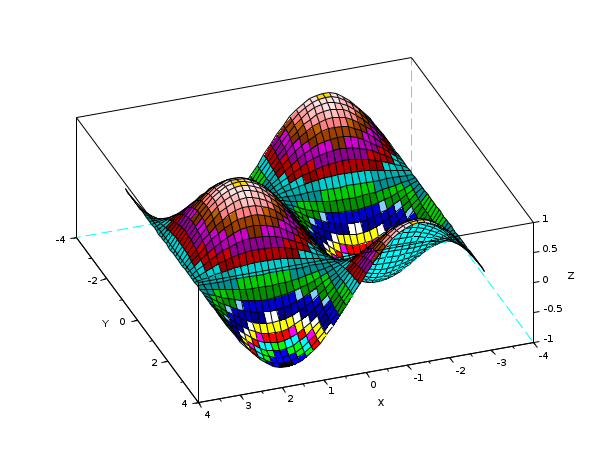Scilab Home page | Wiki | Bug tracker | Forge | Mailing list archives | ATOMS | File exchange
Change language to: English - Português - 日本語 - Русский

See the recommended documentation of this function

Aide de Scilab >> Graphiques > Gestion des couleurs > colormap

# colormap

using colormaps

### Description

A colormap `cmap` is defined by a m x 3 matrix. m is the number of colors. Color number i is given as a 3-uple `cmap(i,1)`, `cmap(i,2)` `cmap(i,3)` corresponding respectively to red, green and blue intensity between 0 and 1.

At the beginning, 32 colors are defined in the colormap. You can change the colormap of a figure by using `set(f,"color_map",cmap)` where `f` is the handle of the figure.

Each color in the colormap has an id you have to use to specify color in most plot functions. To see the ids, use function getcolor.

The functions hotcolormap, jetcolormap and graycolormap provide colormaps with continuous variation of the colors.

You can get the default colormap by `cmap=get(sdf(),"color_map")`.

### Examples

```n=64;
r=linspace(0,1,n)';
g=linspace(1,0,n)';
b=ones(r);
cmap=[r g b];
f=gcf(); f.color_map=cmap;
plot3d1()
f.color_map=get(sdf(),"color_map");```